Home Practice
For learners and parents For teachers and schools
Textbooks
Mathematics
JSS 1 JSS 2 JSS 3
Full catalogue
Pricing SupportLog in

We think you are located in Nigeria. Is this correct?

# Test yourself now

High marks in science are the key to your success and future plans. Test yourself and learn more on Siyavula Practice.

# Chapter 13: Bearing

Bearing is a way of describing one object's position in relation to another object. It describes an object's position using the points of a compass.

Ships use bearing so the sailors know the exact position of a lighthouse or the harbour in relation to where they are. Aeroplanes use bearing so the pilots know the exact position of the airfield, or which direction they must fly. You could use bearing to describe the exact position of your school in relation to your home.

The photograph below shows a real compass that people use to find bearings. In this chapter, you will find bearings using diagrams of a compass.This compass is a different type of compass from the one you have used when doing constructions.

The description has three rules, which you will learn about in this chapter:

• Bearings are measured from the North line.
• Bearings are measured in a clockwise direction.
• Bearings are written as numbers with three digits and a degree symbol.

If you follow these three rules, you can describe the exact position of an object, and there will be no confusion.

## 13.1 Cardinal points

When you use a map, or describe the position of a city or a state, for example, you can use bearings to give an indication of the the direction in which someone would need to travel to get from one place to another. These directions are based on the compass points North ( $N$), South ( $S$), West ( $W$) and East ( $E$).

If you look at a map of Nigeria, you will notice that:

• Katsina is north of Kaduna
• Gombe is south of Jobe
• Kogi is west of Benue
• Edo is east of Ondo.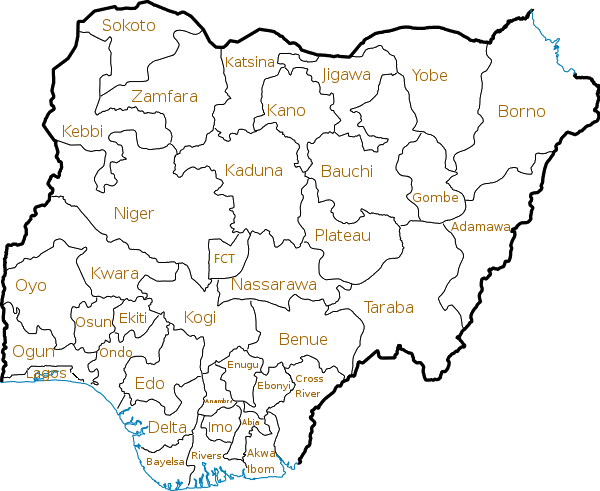The four directions North, South, West and East are known as the major cardinal points.

As the diagram below shows:

• North and South are in opposite directions on a vertical line
• West and East are in opposite directions on a horizontal line
• The North-South line is perpendicular to the West-East line.We can add two more lines to the diagram to include the minor cardinal points:

• North-East ( $NE$) is midway between North and East
• South-East ( $SE$) is midway between South and East
• North-West ( $NW$) is midway between North and West
• South-West ( $SW$) is midway between South and West.In working with bearing, you will need to measure angles from these cardinal points.

When you measure the angles, you will measure in a clockwise direction.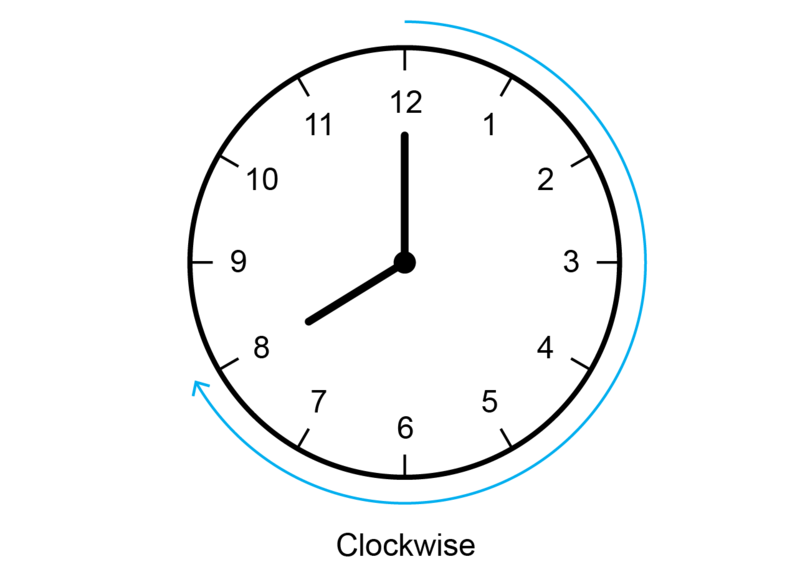To measure the angles, you need to begin at the North line, which is at $0^{\circ}$. North is also at $360^{\circ}$, because a full turn around the compass, which is called a revolution, is equal to $360^{\circ}$.major cardinal points The major cardinal points are North, South, West and East.

minor cardinal points The minor cardinal points are North-East, South-East, North-West and South-West.

clockwise direction The direction in which the hands of a clock (or watch) normally travel is the clockwise direction.

revolution A revolution is one full turn. A revolution is equal to $360^{\circ}$.

### Worked example 13.1: Working with the cardinal points

Using this diagram of a compass, answer the questions that follow.1. Which cardinal point is opposite West?
2. Which cardinal point is opposite North-East?
3. At what angle do you find South?
4. What cardinal point is at an angle of $315^{\circ}$ from North?
1. Step 1: Refer to the diagram to identify West, and find what is opposite.

East is opposite West.

2. Step 2: Refer to the diagram to identify North-East, and find what is opposite.

South-West is opposite North-East.

3. Step 3: Refer to the diagram and identify South.

South is at $180^{\circ}$.

4. Step 4: Refer to the diagram and identify $315^{\circ}$.

North-West is at an angle of $315^{\circ}$ from North.

### Exercise 13.1: Work with the cardinal points

1. Use the map of the provinces of Nigeria to fill in the missing directions.1. Yobe is â€¦. of Gombe.

north

1. Katsina is â€¦ of Zamfara.

east

1. Ogun is â€¦. of Ondo.

west

1. Delta is â€¦ of Edo.

south

2. Draw your own diagram to show the four major cardinal points.3. Use your answer to Question 2. Add two more lines so that the diagram also shows the four minor cardinal points.4. Which cardinal point is opposite East?

West is opposite East.

5. Which cardinal point is opposite North-West?

South-East is opposite North-West.

6. At what angle do you find North-East?

North-East is at $45^{\circ}$.

7. What cardinal point is at an angle of $180^{\circ}$ from North?

South is at an angle of $180^{\circ}$ from North.

## 13.2 Finding bearings

Bearings are measured:

• from the North line
• in a clockwise direction
• and the answers are written as numbers with three digits.

### Worked example 13.2: Finding a bearing

Find the bearing of $B$ from $A$.1. Step 1: Find the North line.

The North line is the vertical line with the label $N$.

2. Step 2: Measure in a clockwise direction.

The size of the angle is given as $40^{\circ}$.

3. Step 3: Give the bearing you have been asked for. Use three digits in the answer.

The bearing of $B$ from $A$ means that you are standing at point $A$ and need to get to $B$. You want to know what angle should be measured at point $A$ so that you can walk in the right direction from $A$ to $B$.

Because $40^{\circ}$ has only two digits, you add a $0$ in front, to show that this is a bearing. You also need to give the degree symbol at the end.

The bearing of $B$ from $A$ is $040^{\circ}$.

It is important to remember that to give a bearing, you always give the angle in a clockwise direction from the North line.

Sometimes you will be given a diagram where the angle is shown in an anti-clockwise direction from the North line. Then you will need to work out what angle to give in your bearing, as shown in the next worked example.

anti-clockwise direction Anti-clockwise is the opposite of clockwise, so it is the direction opposite to that in which the hands of a clock (or watch) normally move.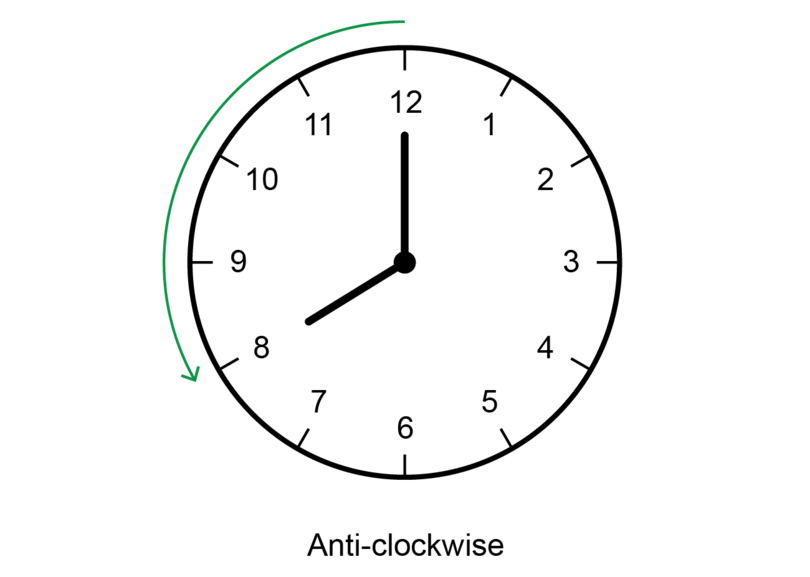### Worked example 13.3: Finding a bearing when angle is given in anti-clockwise direction

Find the bearing of $F$ from $E$.1. Step 1: Find the North line.

The North line is the vertical line with label $N$.

2. Step 2: Convert the given angle to an angle measured in a clockwise direction.

An angle of $72^{\circ}$ is given. This angle is measured in an anti-clockwise direction from the North line.

To find what that angle would be if it was measured in a clockwise direction, you subtract the given angle from $360^{\circ}$.

\begin {align} \text{Clockwise angle} &= 360^{\circ} - 72^{\circ} (\text{revolution}) \\ & = 288^{\circ} \\ \end {align}
3. Step 3: Use three digits in the answer.

The bearing of $F$ from $E$ is $288^{\circ}$.

Notice that in the worked example above:

• The point that has "from" in front of it is always your starting point. This example has "from $E$". You want to find what bearing you must use to get from that point, $E$ to the other point, $F$. So you are asked for "the bearing of $F$ from $E$
• The reason "revolution" is given in brackets. As in geometry, you need to give reasons for your statements when determining bearings.

### Worked example 13.4: Finding a bearing using a straight angle

Find the bearing of $C$ from $D$.1. Step 1: Find the North line.

The North line is the vertical line with label $N$.

2. Step 2: Look carefully at how the given angle is marked.

An angle of $50^{\circ}$ is given, but this angle is not measured from the North line.

3. Step 3: Determine what the angle would be if it was measured clockwise from the North line.

The angle is measured from the South line. The North-South line is a straight line. Remember that a straight line (or a straight angle) is equal to $180^{\circ}$.

To find the size of the angle from the North line, you have to add $180^{\circ}$ to the given angle. Notice how this reason is given in the working below.

\begin {align} \text{Angle that gives bearing} & = 180^{\circ} + 50^{\circ} (\text{str } \angle) \\ & = 230^{\circ} \\ \end {align}4. Step 4: Give the answer, using three digits for the bearing.

The bearing of $C$ from $D$ is $230^{\circ}$.

It is possible to have more than one North line in a diagram.

Worked example 13.5 will show you how to reason if you are given a diagram with more than one North line.

The important thing to remember is that all North lines are parallel to each other. So you use the properties of parallel lines.

When two parallel lines are cut by a transversal line, the co-interior angles are supplementary. This means the co-interior angles add up to $180^{\circ}$.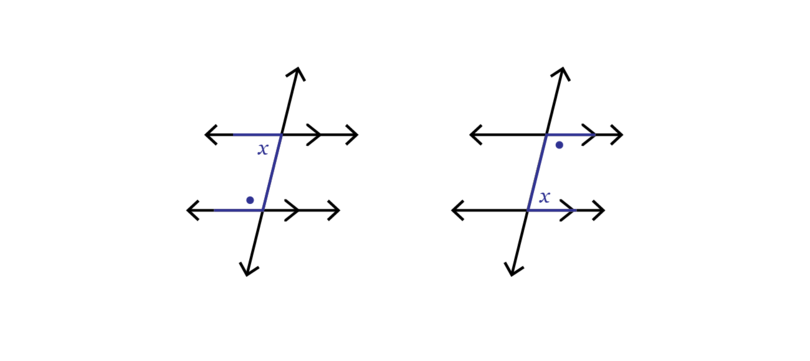### Worked example 13.5: Finding bearings when given two North lines

Using the diagram given below, find the bearing of $B$ from $A$. Then then find the bearing of $A$ from $B$.1. Step 1: Find the bearing of $B$ from $A$ first.

The diagram shows an angle of $60^{\circ}$ measured in a clockwise direction from the North line at point $A$.

2. Step 2: Give the answer, using three digits for the bearing.

The bearing of $B$ from $A$ is $060^{\circ}$.

3. Step 3: Now find the bearing of $A$ from $B$.

There is a second North line drawn from point $B$.

Line $AB$ is now a transversal cutting through two parallel lines (the two North lines).

$A$ and $B$ are co-interior $\angle$ s, so they are supplementary.

4. Step 4: Find the size of the co-interior angle.

\begin {align}\\ \text{Angle at point } B & = 180^{\circ} - 60^{\circ} (\text{co-int } \angle \text{s suppl; North lines are} \parallel)\\ & = 120^{\circ} \\ \end {align}5. Step 5: Find the angle measured clockwise from the North line at $B$.

To find the bearing of $A$ from $B$, you need to measure from the North line at $B$. The angle of $120^{\circ}$ is measured anti-clockwise from this North line.

To find the the angle from the North line, subtract $120^{\circ}$ from $360^{\circ}$.

\begin {align}\\\ \text{Angle that gives bearing} & = 360^{\circ} - 120^{\circ} (\text{revolution})\\\ & = 240^{\circ} \\\ \end {align}6. Step 6: Give the answer, using three digits for the bearing.

The bearing of $A$ from $B$ is $240^{\circ}$.

### Exercise 13.2: Find bearings

1. For each diagram, write down the three digit bearing.

Write the answer as follows: The bearing of â€¦ from â€¦ is â€¦• The bearing of $B$ from $A$ is $026^{\circ}$
• The bearing of $D$ from $C$ is $090^{\circ}$
• The bearing of $F$ from $E$ = $180^{\circ} + 30^{\circ} (\text{str } \angle)$ = $210^{\circ}$
• The bearing of $H$ from $G$ = $360^{\circ} - 90^{\circ} (\text{revolution})$ = $270^{\circ}$
• The bearing of $L$ from $K$ = $60^{\circ} - 48^{\circ} (\text{revolution})$ = $312^{\circ}$
2. Use the diagram below to find the bearing of $Y$ from $X$, and the bearing of $X$ from $Y$.The bearing of $Y$ from $X$ is $058^{\circ}$.

To find the bearing of $X$ from $Y$, use the fact that the two North lines are parallel, so the co-interior angles are supplementary.

\begin {align}\\ \text{Co-interior angle at } Y &= 180^{\circ} - 58^{\circ} (\text{co-int } \angle \text{s suppl; North lines }\parallel)\\ &= 122^{\circ}\\ \end {align}

The angle at $Y$ is measured in an anti-clockwise direction.

\begin {align}\\ \text{Angle measured clockwise at } Y & = 360^{\circ} - 122^{\circ} (\text{revolution})\\ &= 238^{\circ} \\ \end {align}

The bearing of $X$ from $Y$ is $238^{\circ}$.3. Use the diagram below to find the bearing of $Q$ from $P$, and the bearing of $P$ from $Q$.The bearing of $Q$ from $P$ is $115^{\circ}$.

To find the bearing of $P$ from $Q$, use the fact that the two North lines are parallel, so the co-interior angles are supplementary.

\begin {align}\\ \text{Co-interior angle at } Q & = 180^{\circ} - 115^{\circ} (\text{co-int } \angle \text{s suppl; North lines } \parallel)\\ &= 65^{\circ} \\ \end {align}

The angle at $Q$ is measured in an anti-clockwise direction.

\begin {align}\\ \text{Angle measured clockwise at } Q & = 360^{\circ} - 65^{\circ} (\text{revolution}) \\ &= 295^{\circ} \\ \end {align}

The bearing of $P$ from $Q$ is = $295^{\circ}$.4. Use the diagram below to find the bearing of $Y$ from $X$, and the bearing of $X$ from $Y$.\begin {align}\\ \text{Co-interior angle at } X & = 180^{\circ} - 140^{\circ} (\text{co-int } \angle \text{s suppl, North lines} \parallel)\\ &= 40^{\circ} \\ \end {align}

The bearing of $Y$ from $X$ is $040^{\circ}$.

\begin {align}\\ \text{Angle measured clockwise at } Y & = 360^{\circ} - 140^{\circ} (\text{revolution}) \\ & = 220^{\circ} \\ \end {align}

The bearing of $X$ from $Y$ is $220^{\circ}$.5. Use the diagram below to find the bearing of $Q$ from $P$, and of $P$ from $Q$.\begin {align}\\ \text{Co-interior angle at } P & = 180^{\circ} - 20^{\circ} (\text{co-int } \angle \text{s suppl, North lines} \parallel)\\ &= 160^{\circ} \\ \end {align}

The bearing of $Q$ from $P$ is $160^{\circ}$.

\begin {align}\\ \text{Angle measured clockwise at } Q &= 360^{\circ} - 20^{\circ} (\text{revolution})\\ & = 340^{\circ} \\ \end {align}

The bearing of $P$ from $Q$ is $340^{\circ}$.## 13.3 Scale drawing and distances

A scale drawing is a diagram in which a real object (for example, a book, a table or a building) is drawn bigger or smaller while keeping the same proportions.

Architects use scale drawings when they design or plan buildings. For example, when you want to build a house you need a floor plan of the house. It is not possible to draw a diagram of the floor plan of a house at its real size, of course. But if you reduce every measurement in the same proportion, then you can fit the diagram onto a sheet of paper.

We can also use a scale drawing together with bearing to show the distances between two objects.

A scale drawing has to show clearly what ratio was used to enlarge or reduce the object. This ratio is called the scale of the drawing.

• A ratio of $1 : 10$ means that 1 unit on the scale drawing represents 10 units in real life. For example, 1 cm on the scale drawing represents 10 cm in real life.
• A ratio of $1 : 700$ means that 1 unit on the scale drawing represents 700 units in real life. For example, 1 mm on the scale drawing represents 700 mm in real life.

scale drawing A scale drawing is a diagram of a real object where every measurement is reduced (or enlarged) in the same proportion.

scale The scale of a scale drawing tells what ratio was used to reduce (or enlarge) the dimensions of the real life object to do the scale drawing.

### Worked example 13.6: Finding a distance using bearing and a scale drawing

On the scale drawing below, the school is at $B$, and the clinic is at $A$.

Describe the exact position of the clinic if a scale of $1 : 500$ was used.1. Step 1: Read off the distance between the school and the clinic on the scale diagram.

The diagram shows a distance of 7 cm.

2. Step 2: Calculate the distance in real life.

\begin {align}\\ \text {Distance on scale drawing} & = \text{7 cm} \\ \therefore \text{Distance in real life} & = \text{7 cm} \times 500 \\ &= \text{3,500 cm}\\ &=\text{35 metres} \end {align}
3. Step 3: Describe the position of the clinic, giving its bearing and distance from the school.

The clinic is 35 metres from the school, at a bearing of $050^{\circ}$.

When working with bearings and scale drawings, you may need to work with the properties of a triangle. If you need to calculate the length of one side of a right-angled triangle, you may use the theorem of Pythagoras.

If $\triangle ABC$ is a right-angled triangle with $\hat {B}$ equal to $90^{\circ}$, the theorem of Pythagoras states: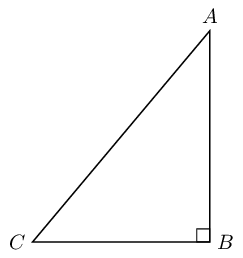For the exercise below, remember that 100 centimetres = 1 metre, and 1,000 metres = 1 kilometre.

### Exercise 13.3: Find distances using bearing and scale drawings

1. The distance between two buildings, $A$ and $B$, is shown on the scale drawing below.What is the distance between the two buildings in real life? Give the answer in metres.

\begin {align}\\ \text {Distance on scale drawing} & = \text{8.35 cm} \\ \therefore \text{Distance in real life} & = \text{8.35 cm} \times 600 \\ &= \text{5,010 cm}\\ &=\text{50.1 metres} \end {align}
2. A football player runs between two cones marked $A$ and $B$ on the diagram.What is the distance that the player covers if she runs from $A$ to $B$ and then back to $A$? Give the answer in metres.

\begin {align} \text {Distance on scale drawing }& = \text{2.25 cm}\\ \therefore \text{Distance in real life } & = \text{2.25 cm} \times 1,000\\ &= \text{2,250 cm}\\ &=\text{22.5 metres}\\ \end {align} \begin {align} \text {Distance from } A \text{ to } B \text{ and then back to } A & = 2 \times 22.5 \text{ metres} \\ & = 45 \text{ metres}\\ \end {align}
3. An athlete trains for an upcoming event by running from his house ( $P$) to work ( $Q$) every day.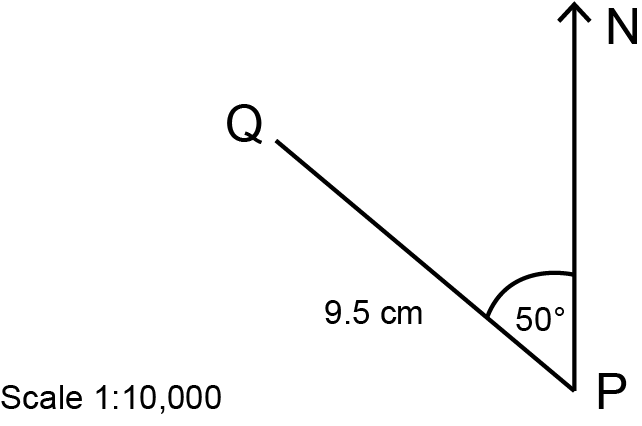Use the scale drawing to describe the bearing and distance of the athlete's daily run. Give the distance in metres.

Bearing:

\begin {align}\\ \text{Angle measured clockwise at } P &= 360^{\circ} - 50^{\circ} (\text{revolution})\\ & = 310^{\circ} \\ \end {align}

The bearing of $Q$ from $P$ is $310^{\circ}$.

Distance:

\begin {align} \text {Distance on scale drawing }& {= \text{9.5 cm}}\\ \therefore \text{Distance in real life } & {= \text{9.5 cm} \times 10,000}\\ &= \text{95,000 cm}\\ &=\text{950 metres} \end {align}

The athlete's daily run is 950 metres from his house to work at a bearing of $310^{\circ}$.

4. Amadi and Chidi go to the same school ( $P$). Amadi's house is at $R$, and Chidi's house is at $Q$. The scale drawing shows the position of the school, as well as the position of the two houses.1. What is the distance in kilometres between the school and Amadi's house?

Distance between the school ( $P$) and Amadi's house ( $R$):

\begin {align} \text {Distance on scale drawing }& = \text{12 cm}\\ \therefore \text{Distance in real life } & = \text{12 cm} \times 10,000\\ &= \text{120,000 cm}\\ &=\text{1,200 metres}\\ &= \text{1.2 km}\\ \end {align}

The distance from the school to Amadi's house is 1.2 kilometres.

1. What is the distance in kilometres between Amadi's house and Chidi's house?

You will need to use the theorem of Pythagoras to find the distance between the two houses on the scale drawing.

\begin {align} QR^2 & = PR^2 + PQ^2 (\text{Pythagoras}) \\ & = 5^2 + 12^2 \\ & = 25 + 144\\ & = 169\\ \therefore QR & = \sqrt {169}\\ & = 13\\ \end {align}

So, the distance between the two houses on the scale drawing is 13 cm.

\begin {align} \text {Distance on scale drawing }& = \text{13 cm}\\ \therefore \text{Distance in real life } & = \text{13 cm} \times 10,000\\ &= \text{130,000 cm}\\ &=\text{1,300 metres}\\ &= \text{1.3 km}\\ \end {align}

The distance between Amadi's house and Chidi's house is 1.3 kilometres.

5. In the diagram, point $A$ shows the position of Town $A$.1. Copy the diagram and complete a scale drawing to show that Town $B$ is at a bearing of $300^{\circ}$ from Town $A$. The distance between the two towns on the scale drawing is 4.5 cm.

Remember that to construct an angle of $300^{\circ}$ in a clockwise direction, you have to subtract the $300^{\circ}$ from $360^{\circ}$. Then you can construct an angle in an anti-clockwise direction from the North line.

Draw an accurate scale drawing, using the following steps:

• Copy the given North line and mark point $A$.
• Use a protractor to mark an angle of $360^{\circ} - 300^{\circ} = 60^{\circ}$ in an anti-clockwise direction.
• Construct $AB$ = 4.5 cm.1. Work out the real distance between the two towns if the scale is $1 : 50,000$. Give your answer in kilometres.
\begin {align} \text {Distance on scale drawing }& = \text{4.5 cm}\\ \therefore \text{Distance in real life } & = \text{4.5 cm} \times 50,000\\ &= \text{225,000 cm}\\ &=\text{2,250 metres}\\ &= \text{2.25 km}\\ \end {align}

The distance between the two towns is 2.25 kilometres.

## 13.4 Summary

• Bearing is a way of describing one object's position in relation to another object.
• There are three rules for giving a description of bearing:
• Bearings are measured from the North line.
• Bearings are measured in a clockwise direction.
• Bearings are written as numbers with three digits and a degree symbol.
• The four directions (North, South, West and East) are known as the major cardinal points.
• There are also four minor cardinal points:
• North-East ( $NE$) is midway between North and East
• South-East ( $SE$) is midway between South and East
• North-West ( $NW$) is midway between North and West
• South-West ( $SW$) is midway between South and West.
• The bearing of $B$ from $A$ means that you are standing at point $A$, and you want to know what angle should be measured at point $A$ so that you can walk in the right direction from $A$ to $B$.
• It is possible to have more than one North line in a diagram.
• All North lines are parallel to each other.
• When two parallel lines are cut by a transversal line, the co-interior angles are supplementary.
• Bearings can be used in a scale drawing to show the distances between two objects.
• A scale drawing is a diagram of a real object where every measurement is reduced (or enlarged) in the same proportion.